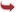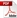International Journal of Scientific and Research Publications

#### IJSRP, Volume 4, Issue 1, January 2014 Edition [ISSN 2250-3153]On The Homogeneous Bi-quadratic Equation with FiveUnknowns x^4-y^4=8(z+w) p^3
M.A.Gopalan, S. Vidhyalakshmi, E.Premalatha
Abstract: The biquadratic equation with 5 unknown given by is analyzed for its patterns of non – zero distinct integral solutions. A few interesting relations between the solutions and special polygonal numbers are exhibited.
[VIEW FULL PAPER][DOWNLOAD]

Reference this Research Paper (copy & paste below code):

M.A.Gopalan, S. Vidhyalakshmi, E.Premalatha (2018); On The Homogeneous Bi-quadratic Equation with FiveUnknowns x^4-y^4=8(z+w) p^3; Int J Sci Res Publ 4(1) (ISSN: 2250-3153). http://www.ijsrp.org/research-paper-0114.php?rp=P252133